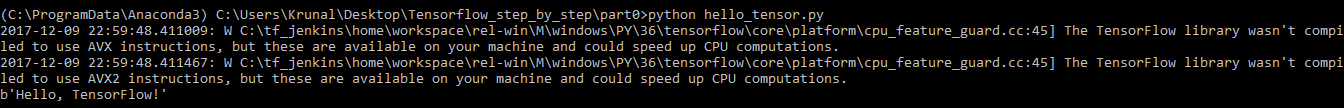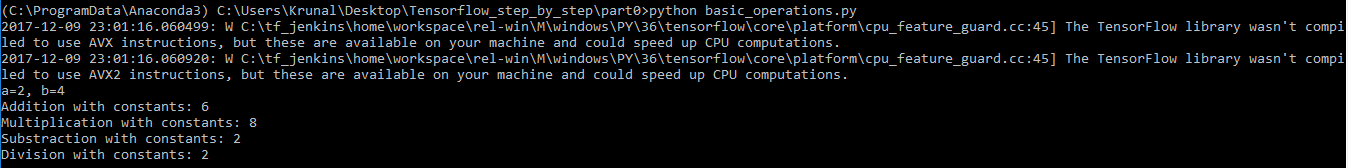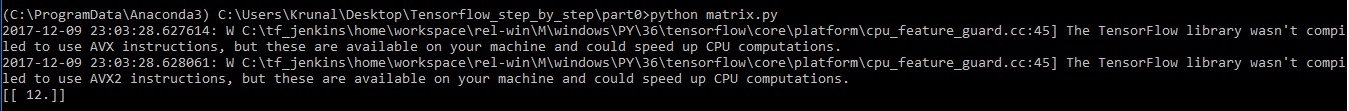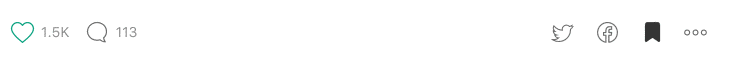### Maths operations in Tensorflow

Fri, Feb 9, 2018 3-minute read

Machine Learning is making a boom in the tech world, specifically for the developers and for the talented data scientists. We will never learn new things if we don’t start it from the bottom. In this article, we are striking at the root of Tensorflow. After reading this article you will be able to perform some basic mathematical operations in Tensorflow.

# Where it starts?

Tensorflow was published in November 2015 by the Google Brain Team and currently Tensorflow 1.5 version is the latest release with Tensorflow lite, announced for mobile and embedded devices.

Tensor is a multidimensional array. Nodes in the graph (aka ops or operations) perform mathematical computations and produce the tensors.

Have you installed Tensorflow on your PC yet? Not yet? Click the link and follow the instructions.

For running the following code you will need:

• Python 3.5
• Tensorflow
• Anaconda IDE

If you are getting errors while installing it, post it on StackOverflow.

To check the current version of Tensorflow run the following code in the command line:

Above code will print the version of Tensorflow.

# Let’s begin it.

I assume that you have correctly installed above listed tools and now activate tensorflow.

## Hello World

Now let’s understand the code, we have imported print_function and tensorflow libraries. Created the constructor of the constant class form tensorflow and added the values to it Hello TensorFlow! In tensorflow session, we have run the node of constant op. That’s it??

As I am running this code in Anaconda command prompt, it is not necessary to activate the Tensorflow for basic operations.

Output:## Arithmetic operations

We have learned to make the constant node and printing the values in it. Next is to performing arithmetic operations.

We have created the 2 constant constructors, assigned the values in a and b. In the Tensorflow session, we are running calculating the operations in it. the values returned by the constants represents the output of the constant ops. Need Explanations in above code….???

Output:In the above code snippet we have added the values at the initialization time, now we are writing the code to perform those operations at the run-time.

We have defined types of variables in tf.placeholder to feed the data when the session is running, by feed_dict we have added data in the Tensorflow session.

## Matrix manipulation

Source giphy.

We have implemented basic arithmetic operations and now time is to the same with the matrix.

We have created two matrices, in matrix1 we have created one 1x2 matrix, in matrix2 we have created a 2x1 matrix. In above code, matmul will return the multiplication of the two matrices.

OutputFrom above we can declare the variables and perform the basic maths operations in it. Run the above code and be confident in it.

The programmer who writes code to learn, learns quickly.

Next step will be to understand maths operations. How mean, median and mode works for the data and in graph.